# Intel Partition Table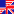English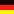Deutsch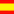Español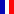Français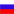Русский

## How is the Partition Table written?

CHS (Cylinder, Head, Sector) values are limited by a set number of bits for each value in the 16-byte partition table entries to: 1023,254,63. So LBA and CHS values can't be equal for HD bigger than 8 GB.

There are two ways to store the CHS value -

• first way;
``` convert LBA to CHS,
store (cylinder & 0x3FF, head & 0xFF, sector & 0x3F)
```

It's what Partition Magic does (prior to version 8.0?).

• second way;
``` convert LBA to CHS
if cylinder <= 1023,
store (cylinder & 0x3FF, head & 0xFF, sector & 0x3F)
else
store (1023, max_head & 0xFF, max_sector & 0x3F)
```

This is what Linux fdisk and TestDisk do.

When TestDisk checks the partition table, it assumes both ways might be correct. But the second way is better because start CHS is always lower or equal to end CHS.

Example: A hard disk's logical geometry is 255 heads per cylinder and 63 sectors per head. A partition begins at LBA=46781280 or CHS=2912,0,1. This partition ends at 3072,254,63.

• First way:
``` start:  864,  0, 1
end:      0,254,63
```
• Second way:
``` start: 1023,254,63
end:   1023,254,63
```

NB: 1023 = 0x3FF (1023*255+254)*63+63-1=16450559 (2912*255+ 0)*63+ 1-1=46781280

Partition Magic (before version 8.0?) considers the second way as invalid even though it's an agreed upon standard. TestDisk handles both without complaining.